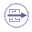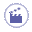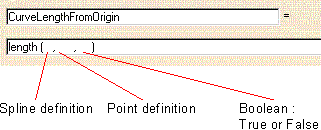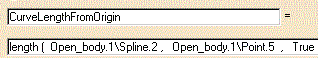# Specifying a Measure in a FormulaThe purpose of this task is to explain how to specify that the value of a Length type parameter is equal to the curved abscissa of a point located on a curve.Measures, i.e. values captured from the geometry area can be used in formulas. Here are some examples of measures that can be used in formulas:
 Distance between two points. Total length of a curve. Length of a curve segment - between a point and the origin or between a point and the curve extremity. Length of a curve segment - between two points. Area of  an extruded surface.1. Open the KwrMeasure.CATPart document. The whole document has been created using the Generative Shape Design product. The Extrude.1 and Extrude.2 surfaces are extruded from the Spline.1 and Spline.2 curves. The point whose abscissa is to be measured is Point.5. The origin of the curve where Point.5 is located on is Point.82. Click the Formula icon. The f(x) dialog box is displayed.

3. Create the CurveLengthFromOrigin parameter.

 Select the Length item with Single Value from the New Parameter of type list, then click New Parameter of type. The new parameter appears in Edit name or value of the current parameter. Replace the Length.1 name with CurveLengthFromOrigin, and click Apply.
4. Specify that the value of CurveLengthFromOrigin is the abscissa of Point.5:

1. Select the CurveLengthFromOrigin parameter from the parameters list, then click Add Formula. The Formula editor is displayed.

2. Select the Measures item from the Dictionary list.

3. From the list of measures, double-click  the length(Curve,Point,Boolean) item. The length function is added to the Formula Editor.4. Fill in the Formula editor field as indicated below.

1. The three arguments are: a curve to be selected from the geometry area, a point to be selected from the geometry area and a boolean.

2. Position the cursor where the first argument is intended to be typed. Then double-click the Spline.2 feature in the specification tree. The curve argument is added to the length definition.

3. Position the cursor where the second argument is intended to be typed. Then double-click the Point.5 feature in the specification tree. The point argument is added to the length definition.

4. Type a boolean for the third argument:  True if  the length is to be calculated from the origin,  False if  the length is to be calculated from the curve end.5. Click OK to confirm the formula definition. You are back to the Formulas dialog box. The CurveLengthFromOrigin formula and value(47.5mm) are added to the parameter list.

5. Click OK to add the parameter as well as its formula to the document.